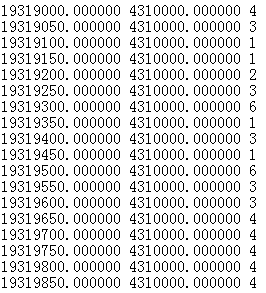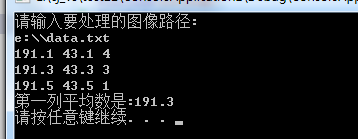c++中如何提取文件每一行的一部分int main()
{
Cluster picture1;

``````cout << "请输入要处理的图像路径:" << endl;

cin >> filename;

file.open(filename);

if (!file.is_open())
{
cout << "有错误！文件未被打开\n";
}
for (int j = 0; j <100; j++)
{
for (int i = 0; i <100; i++)
if (!file.eof())
{
getline(file, line);//按行提取文件（以图像左下角开始，从左向右，从下到上遍历）
int size = line.length();
char a = line[size - 1];//取出每行中第32个字符
picture1.set_tu(i, j, a);//把数字存储到数组中
}
}
``````

4个回答

#include
#include
#include
#include
#include
using namespace std;

vector vec_num;
int main()
{
string filename,line;
cout << "请输入要处理的图像路径:" << endl;

``````cin >> filename;
ifstream file;
file.open(filename);

if (!file.is_open())
{
cout << "有错误！文件未被打开\n";
}

while(getline(file, line))
{
float first_num,second_num;
int third_num;
sscanf(line.c_str(), "%f %f %d", &first_num, &second_num, &third_num);//格式化提取
cout << first_num << " " << second_num << " " << third_num << endl;//打印一下数据
//这里假设你把第一列的数据存下来,我这里是用vector存的
//如果你可能三列都要用可以弄个结构体vector<MyStruct> vec_num;
vec_num.push_back(first_num);
}

float avg_num = 0.0;//保存计算出来的平均数结果
//遍历vector
vector<float>::iterator it;
for(it = vec_num.begin(); it != vec_num.end(); it++)
{
avg_num += *it;
}
avg_num = avg_num / vec_num.size();
cout << "第一列平均数是:" << avg_num << endl;

system("pause");
return 0;
``````

}4 年多之前 回复

for (int j = 0; j <100; j++)
{
for (int i = 0; i <100; i++)
if (!file.eof())
{
getline(file, line);//按行提取文件（以图像左下角开始，从左向右，从下到上遍历）
int size = line.length();
//得到最后一个数字可以这样读取
int count=0;
for(int t=0;t<line.size;t++)
{
if(line[size]==' ')//判断是空格
{
count+=1;
if(count==3)
{
char[]st1;
for(int tt=t+1;tt<size;tt++)
{
strcat(st1,line[size]);
}

``````                        break;
}
}
}
char a = line[size - 1];//取出每行中第32个字符
picture1.set_tu(i, j, a);//把数字存储到数组中
}
``````

}
//然后写个函数吧数组转化成数字就可以了，代码我就不写了。